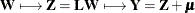### Unconditional Simulation

It is a simple matter to produce an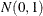random number, and by stacking k such numbers in a column vector you obtain a vector with independent standard normal components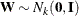. The meaning of the terms independence and randomness in the context of a deterministic algorithm required for the generation of these numbers is somewhat subtle; see Knuth (1973, Vol. 2, Chapter 3) for a discussion of these issues.

Rather than, what is required is the generation of a vector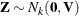—that is,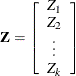with covariance matrix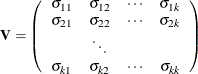where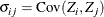If the covariance matrix is symmetric and positive definite, it has a Cholesky rootsuch that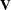can be factored as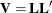whereis lower triangular. See Ralston and Rabinowitz (1978, Chapter 9, Section 3-3) for details. This vector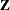can be generated by the transformation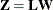. Note that this is where the assumption of multivariate normality is crucial. If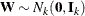, thenis also normal or Gaussian. The mean of Z is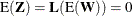and the variance is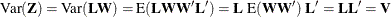Finally, let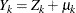; that is, you add a mean term to each variable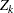. The covariance structure of the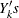remains the same. Unconditional simulation is done by simply repeatedly generating krandom numbers, stacking them, and performing the transformation